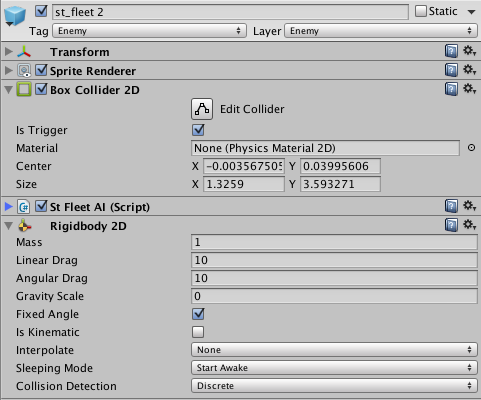# Collision Detection Between Instances of Prefabs doesn't work

I have a problem, that may have a simple solution. I’m making a 2D game and I have enemies that spawn and follow a player. Everything works fine, except that when 2 enemies should “repel” each other they just overlap.

I have a script with the AI and I’m manipulating the rigidbodies to move the enemies because I read that it should fix the problem, but it didn’t.

Here is the AI code

``````using UnityEngine;
using System.Collections;

public class StFleetAI : MonoBehaviour {

Transform target;
public float rotationSpeed;
Transform myTransform;

void Start()
{
myTransform = transform;

}

void RotateToPlayer()
{
float x = target.position.x - myTransform.position.x;
float y = target.position.y - myTransform.position.y;
float angle = Mathf.Atan2(x, y) * Mathf.Rad2Deg;
Quaternion q = Quaternion.Euler(0,0,-angle);
myTransform.rotation = Quaternion.Slerp(myTransform.rotation,q, rotationSpeed*Time.deltaTime);
}

void Update () {
target = GameObject.FindWithTag("Player").transform;
RotateToPlayer();
restrictMovement();

}

void restrictMovement()
{
if (transform.position.x <= -830) {
transform.position = new Vector3(-830, transform.position.y, transform.position.z);
} else if (transform.position.x >= 830) {
transform.position = new Vector3(830, transform.position.y, transform.position.z);
}

if (transform.position.y <= -535) {
transform.position = new Vector3(transform.position.x, -535, transform.position.z);
} else if (transform.position.y >= 535) {
transform.position = new Vector3(transform.position.x, 535, transform.position.z);
}
}

}
``````

Here is the enemy’s setupI’m not sure if the physics engine works the collisions automatically or if I should use a separate algorithm.

Any help would be awesome, thanks!

The isTrigger has to be unchecked.